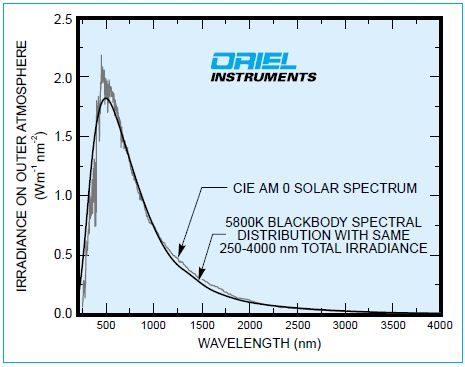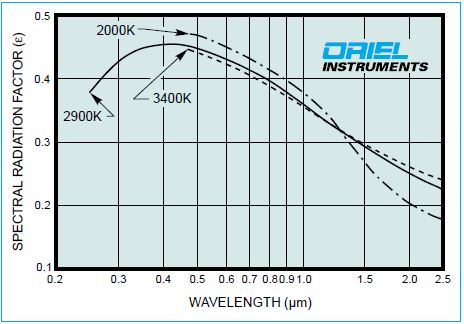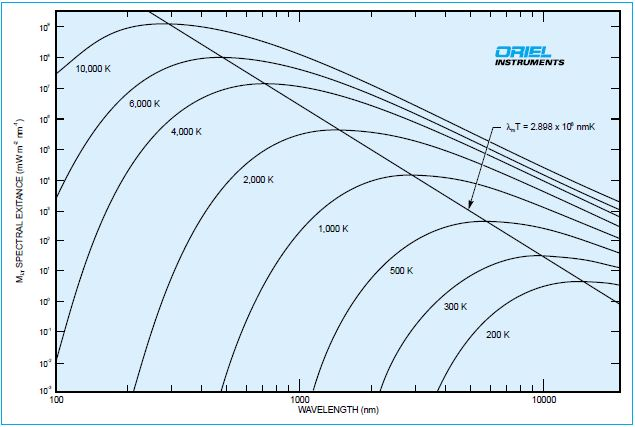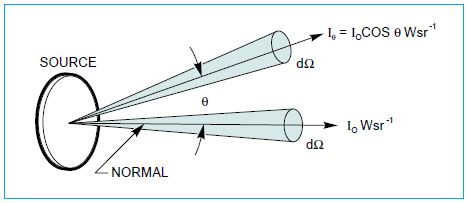# Introduction to the Laws of Electro-Magnetic Radiation

Electromagnetic radiation is radiated and absorbed by all objects. Many important radiation laws are based on the performance of a perfect steady state emitter known as a black body or full radiator. These have smoothly varying spectra that follow a set of laws relating the spectral distribution and total output to the temperature of the blackbody. Sources such as the sun, tungsten filaments, or our Infrared Emitters, have blackbody-like emission spectra. As seen in Figure 1, the spectral distributions of these differ from those of true black bodies as they have slightly different spectral shapes and in the case of the sun, fine spectral detail.Figure 1. The spectrum of radiation from the sun is similar to that from a 5800K blackbody.

## Planck’s Law

Planck’s law gives the spectral distribution of radiant energy inside a blackbody.

W (λ,T) = 8πhcλ-5 (ech/kλT-1) -1

Where:

T = Absolute temperature of the blackbody h = Planck's constant (6.626 x 10-34 Js) c = Speed of light (2.998 x 108 m s-1) k = Boltzmann's constant (1.381 x 10-23 JK-1) λ = Wavelength in m

The spectral radiant exitance from a non perturbing aperture in the blackbody cavity, M (λ,T), is given by:

M (λ,T) = (c/4)W (λ,T),

L (λ,T), the spectral radiance at the aperture is given by:

L (λ,T) = (c/4π)W (λ,T)

The curves in Figure 3 show M plotted for blackbodies at various temperatures. There is an increase in output and the peak shifts to shorter wavelengths as the temperature T increases.

## Stefan-Boltzman Law

By integrating the spectral radiant exitance over all wavelengths,

∫ M (λ,T)dλ = Me (T) = σT4

Where σ is called the Stefan-Boltzmann constant

This is the Stefan-Boltzmann law relating the total output to temperature.

If Me (T) is in W m-2, and T in kelvins, then σ is 5.67 x 10-8 Wm-2 K-4.

At room temperature a 1 mm2 blackbody emits about 0.5 mW into a hemisphere. At 3200 K, the temperature of the hottest tungsten filaments, the 1 mm2, emits 6 W.

## Wien Displacement Law

This law relates the wavelength of peak exitance, λm, and blackbody temperature,T:

λmT = 2898 where T is in kelvins and λm is in micrometers.

For a blackbody at room temperature, the peak of the spectral distribution curve is at 9.8µm. As the source temperature gets higher, the wavelength of peak exitance moves towards shorter wavelengths. The temperature of the sun's surface is around 5800K. The peak of a 6000 blackbody curve is at 0.48µm, as shown in Figure 3.

## Emissivity

Radiation actually obtained is always less than that of a blackbody. Emissivity (ε) is a measure of how a real source compares with a blackbody. It is defined as the ratio of the radiant power emitted per area to the radiant power emitted by a blackbody per area. Emissivity can be wavelength and temperature dependent as seen in Figure 2. As the emissivity of tungsten is less than 0.4 where a 3200 K blackbody curve peaks, the 1 mm2 tungsten surface at 3200 K will only emit 2.5 W into the hemisphere.

If the emissivity does not vary with wavelength then the source is a "graybody".Figure 2. Emissivity (spectral radiation factor) of tungsten.Figure 3. Spectral exitance for various blackbodies

## KIRCHOFF'S LAW

Kirchoffs Law states that the emissivity of a surface is equal to its absorptance, where the absorptance (α) of a surface is the ratio of the radiant power absorbed to the radiant power incident on the surface.

T α(λ,T)dλ = ∫T ε(λ,T)dλ

α = ε

## Lambert’s Law

Lambert's Cosine Law holds that the radiation per unit solid angle (the radiant intensity) from a flat surface varies with the cosine of the angle to the surface normal as seen in Figure 4. Some Oriel Sources, such as arcs, are basically spherical. These appear like a uniform flat disk as a result of the cosine law. Another result of this law is that flat sources, such as some of our low power quartz tungsten halogen filaments, must be properly oriented for maximum irradiance of a target. Flat diffusing surfaces are said to be ideal diffusers or Lambertian if the geometrical distribution of radiation from the surfaces obeys Lambert's Law. Lambert's Law has very important consequences in the measurement of light. Cosine receptors on detectors are needed to make meaningful measurements of radiation with large or uncertain angular distribution.Figure 4. Lamberts cosine law indicates how the intensity, I,depends on angle.This information has been sourced, reviewed and adapted from materials provided by Oriel Instruments.

## Citations

• APA

Oriel Instruments. (2019, July 25). Introduction to the Laws of Electro-Magnetic Radiation. AZoOptics. Retrieved on July 03, 2020 from https://www.azooptics.com/Article.aspx?ArticleID=828.

• MLA

Oriel Instruments. "Introduction to the Laws of Electro-Magnetic Radiation". AZoOptics. 03 July 2020. <https://www.azooptics.com/Article.aspx?ArticleID=828>.

• Chicago

Oriel Instruments. "Introduction to the Laws of Electro-Magnetic Radiation". AZoOptics. https://www.azooptics.com/Article.aspx?ArticleID=828. (accessed July 03, 2020).

• Harvard

Oriel Instruments. 2019. Introduction to the Laws of Electro-Magnetic Radiation. AZoOptics, viewed 03 July 2020, https://www.azooptics.com/Article.aspx?ArticleID=828.